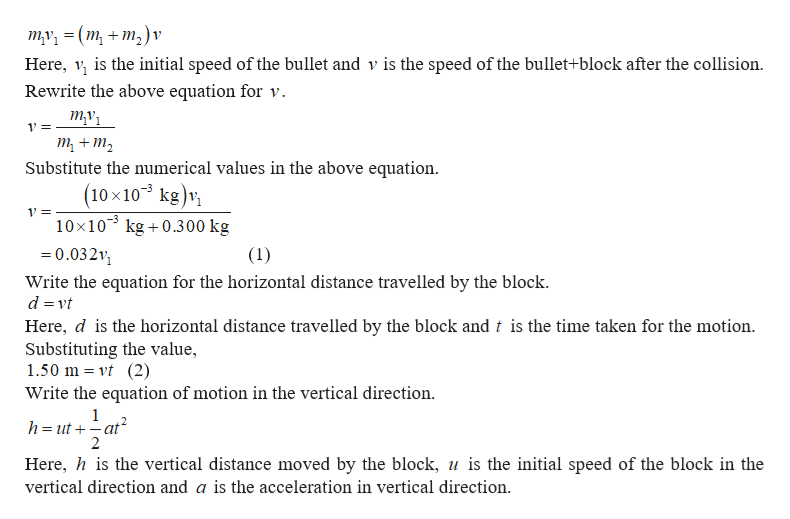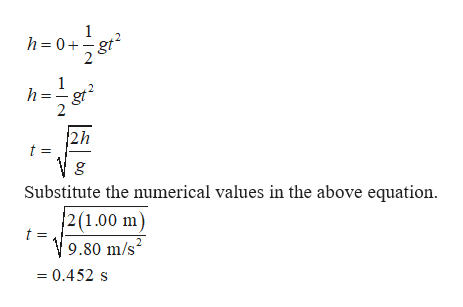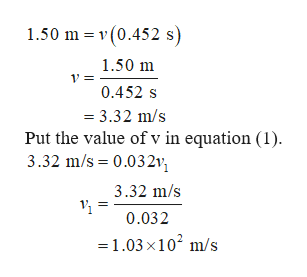# BlockBulletTable1.00 mFloor1.50 m

Question

A bullet of mass m1 = 10.00 g that has a velocity of v1 in the horizontal direction hits and gets fully stuck inside a block of mass m2 of 0.300 kg. That mass m2 is at the edge of a table 1.00 m above the level of the floor. After the bullet gets stuck at the block, that block does a projectile motion and lands 1.50 m in the horizontal direction to the right as shown in the figure. Ignoring air drag calculate the initial speed of the bullet.

a. 2.27 ×102 m/s
b. 1.03 ×102 m/s
c. 3.35 ×102 m/s
d. 1.38 ×101 m/s

check_circleExpert Solution
Step 1

The total momentum of the system must be conserved. The initial momentum of the system is equal to the initial momentum of the bullet since initially the block is at rest. The conservation of linear momentum gives,help_outlineImage Transcriptioncloseту, %3D (m, + m,)v Here, is the initial speed of the bullet and v is the speed of the bullet+block after the collision. Rewrite the above equation for v ту 1= т + т, Substitute the numerical values in the above equation (10x103 kg)v 10x103 kg 0.300 kg 0.03211 (1) Write the equation for the horizontal distance travelled by the block d vt Here, d is the horizontal distance travelled by the block and t is the time taken for the motion Substituting the value, 1.50 m vt (2) Write the equation of motion in the vertical direction 1 h=utat 2 Here, his the vertical distance moved by the block, u is the initial speed of the block in the vertical direction and a is the acceleration in vertical direction fullscreen
Step 2

The initial vertical speed of the block is zero and taking the downward direction to be positive, the value of acceleration will be acceleration due to gravity.help_outlineImage Transcriptioncloseh=0+gt h=g gt2 2h t = g Substitute the numerical values in the above equation. 2(1.00 m) 9.80 m/s = 0.452 s fullscreen
Step 3

Substitute the value of t...help_outlineImage Transcriptionclose1.50 m v (0.452 s) 1.50 m 0.452 s 3.32 m/s Put the value of v in equation (1) 3.32 m/s 0.032v 3.32 m/s 0.032 =1.03 x102 m/s fullscreen

### Want to see the full answer?

See Solution

#### Want to see this answer and more?

Solutions are written by subject experts who are available 24/7. Questions are typically answered within 1 hour*

See Solution
*Response times may vary by subject and question
Tagged in

### Kinematics